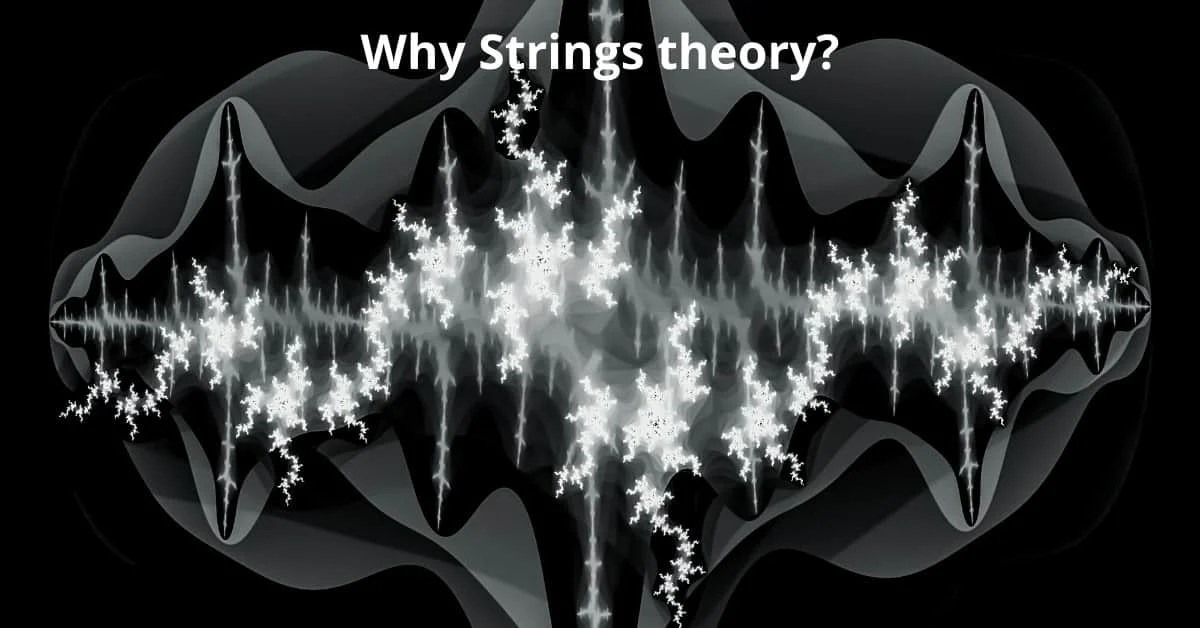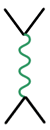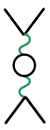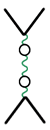# Why String theory?Once special relativity was on the firm observational and theoretical footing, it was appreciated that the Schrödinger equation of quantum mechanics was not Lorentz invariant, therefore quantum mechanics as it was so successfully developed in the 1920s was not a reliable description of nature when the system contained particles that would move at or near the speed of light.

The problem is that the Schrödinger equation is first order in time derivatives but second order in spatial derivatives. The Klein-Gordon equation is second order in both time and space and has solutions representing particles with spin 0:

Dirac came up with “square root” of Klein-Gordon equation using matrices called “gamma matrices”, and the solutions turned out to be particles of spin 1/2:

where the matrix hmn is the metric of flat spacetime. But the problem with relativistic quantum mechanics is that the solutions of the Dirac and Klein-Gordon equation have instabilities that turn out to represent the creation and annihilation of virtual particles from essentially empty space.

Further understanding led to the development of relativistic quantum field theory, beginning with quantum electrodynamics, or QED for short, pioneered by Feynman, Schwinger and Tomonaga in the 1940s. In quantum field theory, the behaviors and properties of elementary particles can be calculated using a series of diagrams, called Feynman diagrams, that properly account for the creation and annihilation of virtual particles.

The set of the Feynman diagrams for the scattering of two electrons looks like+++ …

The straight black lines represent electrons. The green wavy line represents a photon, or in classical terms, the electromagnetic field between the two electrons that makes them repel one another. Each small black loop represents a photon creating an electron and a positron, which then annihilate one another and produce a photon, in what is called a virtual process. The full scattering amplitude is the sum of all contributions from all possible loops of photons, electrons, positrons, and other available particles.

The quantum loop calculation comes with a very big problem. In order to properly account for all virtual processes in the loops, one must integrate over all possible values of momentum, from zero momentum to infinite momentum. But these loop integrals for an particle of spin J in D dimensions take the approximate form

If the quantity 4J + D – 8 is negative, then the integral behaves fine for infinite momentum (or zero wavelength, by the de Broglie relation.) If this quantity is zero or positive, then the integral takes an infinite value, and the whole theory threatens to make no sense because the calculations just give infinite answers.

The world that we see has D=4, and the photon has spin J=1. So for the case of electron-electron scattering, these loop integrals can still take infinite values. But the integrals go to infinity very slowly, like the logarithm of momentum, and it turns out that in this case, the theory can be renormalized so that the infinities can be absorbed into a redefinition of a small number of parameters in the theory, such as the mass and charge of the electron.

Quantum electrodynamics was a renormalizable theory, and by 1940s, this was regarded as a solved relativistic quantum theory. But the other known particle forces — the weak nuclear force that makes radioactivity, the strong nuclear force that hold neurons and protons together, and the gravitational force that holds us on the earth — weren’t so quickly conquered by theoretical physics.

In the 1960s, particle physicists reached towards something called a dual resonance model in an attempt to describe the strong nuclear force. The dual model was never that successful at describing particles, but it was understood by 1970 that the dual models were actually quantum theories of relativistic vibrating strings and displayed very intriguing mathematical behavior. Dual models came to be called string theory as a result.

But in 1971, a new type of quantum field theory came on the scene that explained the weak nuclear force by uniting it with electromagnetism into electroweak theory, and it was shown to be renormalizable. Then similar wisdom was applied to the strong nuclear force to yield quantum chromodynamics, or QCD, and this theory was also renormalizable.

Which left one force — gravity — that couldn’t be turned into a renormalizable field theory no matter how hard anyone tried. One big problem was that classical gravitational waves carry spin J=2, so one should assume that a graviton, the quantum particle that carries the gravitational force, has spin J=2. But for J=2, 4 J – 8 + D = D, and so for D=4, the loop integral for the gravitational force would become infinite like the fourth power of momentum, as the momentum in the loop become infinite.

And that was just hard cheese for particle physicists, and for many years the best people worked on quantum gravity to no avail.

But the string theory that was once proposed for the strong interactions contained a massless particle with spin J=2.

In 1974 the question finally was asked: could string theory be a theory of quantum gravity?

The possible advantage of string theory is that the analog of a Feynman diagram in string theory is a two-dimensional smooth surface, and the loop integrals over such a smooth surface lack the zero-distance, infinite momentum problems of the integrals over particle loops.

In string theory infinite momentum does not even mean zero distance, because for strings, the relationship between distance and momentum is roughly like

The parameter a’ (pronounced alpha prime) is related to the string tension, the fundamental parameter of string theory, by the relation

The above relation implies a minimum observable length for a quantum string theory of

The zero-distance behavior which is so problematic in quantum field theory becomes irrelevant in string theories, and this makes string theory very attractive as a theory of quantum gravity.

If string theory is a theory of quantum gravity, then this minimum length scale should be at least the size of the Planck length, which is the length scale made by the combination of Newton’s constant, the speed of light and Planck’s constant

although as we shall see later, the question of length scales in string theory is complicated by string duality, which can relate two theories with seemingly different length scales.

Recommended books:

1. Something Deeply Hidden: Quantum Worlds and the Emergence of Spacetime

Buy on Amazon- https://amzn.to/3osXuT62. Introduction To Black Holes, Information And The String Theory Revolution, An: The Holographic Universe

Buy on Amazon- https://amzn.to/35n42Le